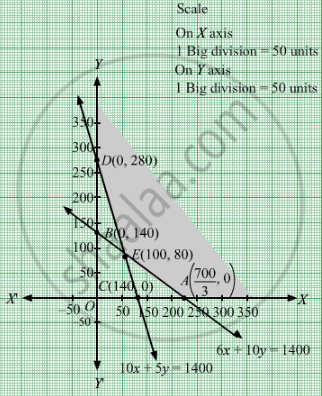# A gardener has supply of fertilizer of type I which consists of 10% nitrogen and 6% phosphoric acid and type II fertilizer which consists of 5% nitrogen and 10% phosphoric acid. - Mathematics

Sum

A gardener has supply of fertilizer of type I which consists of 10% nitrogen and 6% phosphoric acid and type II fertilizer which consists of 5% nitrogen and 10% phosphoric acid. After testing the soil conditions, he finds that he needs at least 14 kg of nitrogen and 14 kg of phosphoric acid for his crop. If the type I fertilizer costs 60 paise per kg and type II fertilizer costs 40 paise per kg, determine how many kilograms of each fertilizer should be used so that nutrient requirements are met at a minimum cost. What is the minimum cost?

#### Solution

Let x kg of type I fertilizer and y kg of type II fertilizer are supplied.
Quantity of fertilizer cannot be negative.
Therefore, $x, y \geq 0$

A gardener has supply of fertilizer of type I which consists of 10% nitrogen and type II fertilizer consists of 5% nitrogen and he needs at least 14 kg of nitrogen for his crop.$\frac{10x}{100} + \frac{5x}{100} \geq 14 \Rightarrow 10x + 5x \geq 1400$

A gardener has supply of fertilizer of type I which consists 6% phosphoric acid and type II fertilizer consists of 10% phosphoric acid.
And he needs  14 kg of phosphoric acid for his crop.

$\frac{6x}{100} + \frac{10x}{100} \geq 14 \Rightarrow 6x + 10x \geq 1400$

Therefore, according to the question, constraints are

$10x + 5y \geq 1400$

$6x + 10y \geq 1400$

If the type I fertilizer costs 60 paise per kg and type II fertilizer costs 40 paise per kg.Therefore, cost of x kg of type I fertilizer and y kg of type II fertilizer is Rs 0.60x and Rs 0.40yrespectively.
Total cost = Z =$0 . 60x + 0 . 40y$  which is to be minimised.
Thus, the mathematical formulat​ion of the given linear programmimg problem is
Min Z =  $0 . 60x + 0 . 40y$subject to
$6x + 10y \geq 1400$
$10x + 5y \geq 1400$
$x, y \geq 0$
First we will convert inequations into equations as follows:
6x + 10y = 1400, 10x + 5y = 1400, x = 0 and y = 0

Region represented by 6x + 10y ≥ 1400:
The line 6x + 10y = 1400 meets the coordinate axes at
$A\left( \frac{700}{3}, 0 \right)$  and B(0, 140) respectively. By joining these points we obtain the line
6x + 10y = 1400. Clearly (0,0) does not satisfies the 6x + 10y = 1400. So, the region which does not contain the origin represents the solution set of the inequation 6x + 10y≥1400.

Region represented by 10x + 5y ≥ 1400:
The line 10x + 5y = 1400 meets the coordinate axes at C(140, 0) and $D\left( 0, 280 \right)$ respectively. By joining these points we obtain the line 10x + 5y = 1400. Clearly (0,0) does not satisfies the inequation 10x + 5y ≥ 1400. So,the region which does not contain the origin represents the solution set of the inequation 10x + 5y ≥ 1400.

Region represented by ≥ 0 and y ≥ 0:
Since, every point in the first quadrant satisfies these inequations. So, the first quadrant is the region represented by the inequations x ≥ 0, and y ≥ 0.
The feasible region determined by the system of constraints 6x + 10y ≥1400, 10x + 5y ≥ 1400, x ≥ 0 and y ≥ 0 are as follows.The corner points are D(0, 280), E(100, 80) and $A\left( \frac{700}{3}, 0 \right)$ The values of Z at these corner points are as follows
 Corner point Z= 0.60x + 0.40y D 112 E 92 A 140

The minimum value of Z is Rs 92 which is attained at E(100, 80).
Thus, the minimum cost is Rs 92 obtained when 100 kg of type I fertilizer and 80 kg of type II fertilizer were supplied.
Concept: Graphical Method of Solving Linear Programming Problems
Is there an error in this question or solution?

#### APPEARS IN

RD Sharma Class 12 Maths
Chapter 30 Linear programming
Exercise 30.4 | Q 23 | Page 53